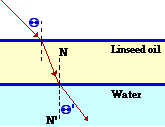# Ray Optics: Refraction and Lenses

## Refraction and Lenses: Audio Guided Solution

#### Problem 8:The diagram at the right shows a ray of light traveling through air towards a thin layer of linseed oil (n = 1.50) resting on top of water (n=1.33). The light ray approaches the linseed oil at an angle of incidence of 48.2°.
a. Determine the angle of refraction at the air-linseed oil boundary.
b. Determine the angle of refraction at the linseed oil-water boundary.

## Habits of an Effective Problem Solver

• Read the problem carefully and develop a mental picture of the physical situation. If necessary, sketch a simple diagram of the physical situation to help you visualize it.
• Identify the known and unknown quantities and record them in an organized manner. Equate given values to the symbols used to represent the corresponding quantity - e.g., do = 24,8 cm; di = 16.7 cm; f = ???.
• Use physics formulas and conceptual reasoning to plot a strategy for solving for the unknown quantity.
• Identify the appropriate formula(s) to use.
• Perform substitutions and algebraic manipulations in order to solve for the unknown quantity.